Mobile QR Code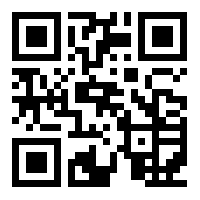1. (Department of Software and Communication Engineering, Sejong Campus, Hongik University, Korea {nadeemali499, jsnb}@hongik.ac.kr )

Intersection management system, Fuzzy logic, Intelligent transport system, Vehicle to vehicle infrastructure

## 1. Introduction

Road accidents can happen anywhere globally, and their probability becomes higher when vehicles cross any intersection point. Multiple factors like careless drivers, unsuitable physical health of the driver, or human error cause such issues . Intelligent transport systems (ITSs) are a future application of next-generation networks like 5G and 6G . An ITS comprises autonomous vehicles and vehicle-to-vehicle (V2V), vehicle-to-infrastructure (V2I), and vehicle-to-pedestrian (V2P) communication. The applications of ITS can be grouped into vehicle-to-everything (V2X) communication .

Autonomous vehicles and intelligent infrastructure have recently gained much recognition as a potential solution for accident avoidance and fatal crashes. Present intersection management system (IMS) techniques are specially created for vehicles supported by infrastructure such as conventional phase-fixed lighting and other more sophisticated adaptive approaches. With the rapid growth of autonomous vehicles (AVs), modern IMS techniques should be established . As V2I communication is a primary feature for the future ITS, it should also be implemented in an IMS.

Robust planning of an IMS is still a big issue. Deciding whom it should put in a queue and which vehicle to allow to move on is still a big issue. How this IMS should decide is a challenge. Computational complexity, efficiency, time, and memory management are big questions for any IMS. In previous years, an ITS has been the subject of interdisciplinary management issues with automated driving and V2I. A multi-agent coordinating and controlling intersection based on crossing asset reserving technology was proposed by Parasumanna Gokulan et al. . When the vehicle approaches a safety zone at a junction, a request for a pass is made, and a decision based on the Early Coming First Operation (ECFO) policy is then made.

A multi-agent-based IMS was proposed by Hamidi et al. . The proposed model consists of two parts. The first part deals with the traffic flow, particularly in emergencies. The second part prevents the overcrowding in paths for a specific time to control congestion situations. A simple heuristic method for resolving the conflicts in space-time when driving a car using Simulation of Urban Mobility (SUMO) was proposed by A. P. Chouhan et al. . A mixed-integer programming intersection coordination algorithm was proposed by Lu et al. to create the fastest route of each vehicle approaching the intersection . A fuzzy logic-based real-time traffic controller was proposed by Nada et al. . The proposed method optimizes the signal setting while taking incoming traffic into consideration.

L. Chen et al. surveyed a cooperative method to deal with intersection management. The author discusses the time slots, space reservation, trajectory planning, and virtual traffic lights in detail . A fuzzy logic-based traffic control signal was proposed by S. Mohanaselvi et al. and deals with unpredictable and linguistic traffic data . Milan Koukol et al. surveyed traffic data management using a fuzzy logic controller for a traffic signal . A fuzzy logic and proportional integration (PI)-based controller was proposed by Tunc et al. for a traffic control system . The study compares the performance of the conventional traffic control signal with the proposed signal. A fuzzy logic-based controller for static road traffic control to minimize traffic jams was proposed by Babangida Zachariah et al. . The proposed model uses real-time traffic data and improves the average waiting time by 65.35 %. E. Namazi presents a comprehensive study on autonomous vehicles and IMS. The author studied both purely autonomous and hybrid autonomous vehicle environments for IMS .

This study's motivation is to use real-time traffic data to automate intersections. The proposed system takes care of emergency vehicles and prioritizes them. A fuzzy inference system is highly adaptable for a complex situation. Fuzzy logic was used to implement local expert knowledge. Local expert knowledge represents the expertise of a person in a specific field. The rule base can only be defined using an expert person's knowledge. In this study, traffic management resources are the local expert knowledge. The rest of the paper is divided into the following parts. Section II shows the problem formulation, section III shows the system model, section IV shows the proposed fuzzy inference system, and section V shows the conclusion.

## 2. Problem Formulation

The main objective of the proposed system is to automate the intersection. The vehicle is equipped with a transceiver module for the proposed model to share the required information. Fig. 1 shows the cross-sectional intersection and all IMSs placed on each leg of the cross-sectional intersection. A circle with a dashed line shows the safety zone. Upon reaching the safety zone, all vehicles share their information to the IMS immediately. The IMS is the first tier of the system. All vehicles share their lane, type, distance from the IMS, and estimated time to reach the IMS. When a vehicle transmits its information, the IMS prompts it to action. These actions are leave,'' standby,'' or stop.'' On the second layer of our system, all the IMSs from all roadsides share their information with a central IMS, known as the CIMS. The CIMS finally decides the action of the vehicles.

##### Fig. 1. Cross-Sectional Intersection.To handle the intersection strategy, we have to follow some assumptions. The assumptions of the systems are as follows.

i. All vehicles are fully autonomous.

ii. Upon reaching the safety zone, the vehicle must ransmit its information to the IMS.

iii. The throughput and latency of communication between the vehicles and the IMS are guaranteed as required.

iv. There is no lane change after reaching the safety zone.

v. All vehicles follow the speed limit and action given by the IMS.

## 3. System Model

The proposed system is based on a two-layer fuzzy inference system. The block diagram of the system is shown in Fig. 2. A vehicle shares its information with the IMS on the first layer (1{\ldots}4). The IMS provides a priority to leave to a vehicle. At this stage, the vehicle gives its information to IMSi about its lane (left, center, right), vehicle type (private, public, emergency), distance from the IMS (low, medium, high), and estimated time to leave the IMS (low, medium, high). Estimated time is used to differentiate the speed of the vehicle. A significant factor is emergency vehicles. All vehicles related to any emergency like ambulances, firefighters, rescue vehicles, and law enforcement are given high priority.

At the second stage, the CIMS prioritizes leaving from all the IMSs. In the case of any conflict, the CIMS allocates the leaving action to the first IMS, the second IMS, the third IMS, and lastly, the fourth IMS. The CIMS makes the final decision.

## 4. Proposed Fuzzy Inference System

Before proceeding further, it is important to look at the functionalities of the fuzzy system. The block diagram in Fig. 3 shows the working mechanism of the fuzzy inference system. In a fuzzy inference system, all relevant factors are collected first. These factors are mapped to crisp inputs using a membership function. The membership function describes the range of factors on the x-axis and the degree of membership on the y-axis.

##### Fig. 3. Hierarchy of Fuzzy Inference System.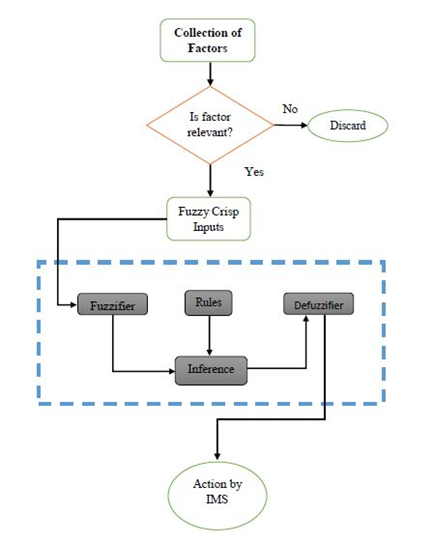Similarly, output membership functions were also defined. Rules were determined based on local expert knowledge, an integral part of the fuzzy system. The local expert knowledge is mainly responsible for output prediction done by the fuzzy system. Based on input parameters, the fuzzy system predicts some output in crisp form. A defuzzifier is used to convert the crisp output to some numeric range. A centroid defuzzifier is used to convert the crisp output to numeric output.

The box with dashed lines shows the working of the fuzzy inference system. The intersection management factors are considered, and the rest is discarded. Then, these factors are modeled with a trapezoidal membership function. The rules were defined based on the relationship between the input and output. For a new input, the fuzzy system predicts some outcome. The output is converted to a semantic range using a defuzzifier. In the proposed model, a Mamdani fuzzy inference system was used .

Table 1 shows the range of all input factors and their ranges. The semantics ranges were mapped according to the given ranges. Table 2 shows the output parameter at layer 1. If the fuzzy system predicts a value of 0-2, the vehicle receives a message to leave. To put a vehicle on standby, the range is 1-4. For a stop signal, the fuzzy system predicts a value of 3-6.

Fig. 4 shows the first layer of the fuzzy inference system, which comprises four variables and one output variable. Each variable contains multiple membership functions, as defined in Table 1. The output parameters are defined in Table 2.

##### Table 1. Input parameters and their rages at layer 1.
 Input Factors Semantic Range Parameters Range Vehicle Lane Right 0-1 Center 0.8-2 Left 1.8-3 Vehicle Type Public Transport 0-1 Private Transport 0.8-2 Emergency Vehicle 1.8-3 Distance From IMS (in meters) Low 0-5 Medium 4-10 High 9-15 Estimate Time (in seconds) Low 0-10 Medium 8-20 High 18-30
##### Table 2. Output parameters at layer 1.
 Output Factor Semantic Range Parameters Range IMS action Leave 0-2 Standby 1-4 Stop 3-6

Fig. 5 shows the second layer of the fuzzy inference system, in which input comes from the first layer, and then the controller decides the output based on the rules. In the second layer, all the IMSs share their information with the CIMS. The input and output memberships are given in Tables 3 and 4, respectively. Table 4 shows the action taken by the CIMS and its ranges.

##### Table 3. Input parameters at layer 2.
 Output Factor Semantic Range Parameters Range IMS1 action Leave 0-2 Standby 1-4 Stop 3-6 IMS2 action Leave 0-2 Standby 1-4 Stop 3-6 IMS3 action Leave 0-2 Standby 1-4 Stop 3-6 IMS4 action Leave 0-2 Standby 1-4 Stop 3-6
##### Table 4. Output parameters at layer 2.
 Output Factor Semantic Range Parameters Range CIMS action Leave IMS1 0-2 Leave IMS2 1-4 Leave IMS3 3-6 Leave IMS4 5-8

### 4.1 Membership Functions

#### 4.1.1 First Layer

In this section, all input parameters and their mathematical relationship are defined. Fig. 6 shows the vehicle lane variable consisting of three membership functions. The line with a circle marker represents the vehicle in the road's right lane. The line with the "x" marker represents the center lane, and the line with the diamond marker represents the left lane. The association of vehicles with the lane is represented by the degree of membership. The degree of the membership function is always defined from 0 to 1. The mathematical form of the vehicle lane variable is given below.

##### Fig. 6. Membership Function for Vehicle Lane.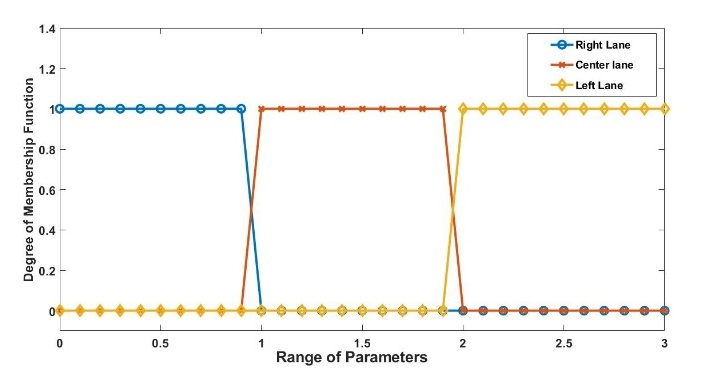In Eq. (1), $\mu _{vl,R}\left(vl\right)$ represents the vehicle in the right lane.

##### (1)
$\begin{equation} \mu _{vl,R}\left(vl\right)=\left\{\max \left(\min \left(1,\frac{1-vl}{0.2}\right),0\right)\right\} \end{equation}$

In Eq. (2), $\mu _{vl,C}\left(vl\right)~$represents the vehicle lane membership function with the center lane.

##### (2)
$\begin{equation} \mu _{vl,C}\left(vl\right)=\left\{\max \left(\min \left(\frac{vl-1}{0.2},1,\frac{1.8-vl}{0.2}\right),0\right)\right\} \end{equation}$

In Eq. (3), $\mu _{vl,L}\left(vl\right)$ represents the vehicle membership function with the left lane.

##### (3)
$\begin{equation} \mu _{vl,L}\left(vl\right)=\left\{\max \left(\min \left(\frac{vl-2}{0.2},1\right),0\right)\right\} \end{equation}$

Fig. 7 shows the vehicle type variable used to distinguish between public transport, private transport, and emergency vehicles. The vehicle type variable is comprised of three membership functions. Public transport is represented using the "o" marker, private transport is expressed with the "x" marker, and emergency vehicles are represented by the "${\lozenge}$" marker.

In Eq. (4), $\mu _{vt,Publictrans}\left(vt\right)$represents the membership function for the public transport vehicle.

##### (4)
$\begin{equation} \mu _{vt,Publictrans}\left(vt\right)=\left\{\max \left(\min \left(1,\frac{1-vt}{0.2}\right),0\right)\right\} \end{equation}$

In Eq. (5), $\mu _{vt,pritrans}\left(vt\right)$ represents the membership function for the private transport vehicle.

##### (5)
$\begin{equation} \mu _{vt,pritrans}\left(vt\right)=\left\{\max \left(\min \left(\frac{vt-1}{0.2},1,\frac{1.8-vt}{0.2}\right),0\right)\right\} \end{equation}$

Eq. (6) shows the membership function of an emergency vehicle. $\mu _{vt,emergency}\left(vt\right)~$represents the emergency vehicle.

##### (6)
$\begin{equation} \mu _{vt,emergency}\left(vt\right)=\left\{\max \left(\min \left(\frac{vt-2}{0.1},1\right),0\right)\right\} \end{equation}$
##### Fig. 7. Vehicle Type Membership Function.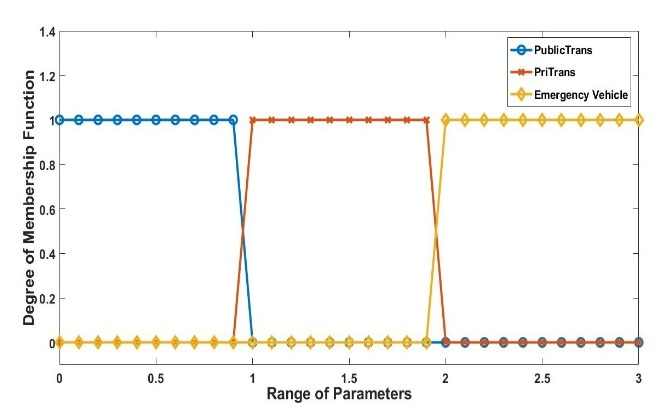Fig. 8 shows the distance from the IMS'' variable. The line with the "o" marker represents a low distance ranging from 0 to 5 meters, and the line with the "x" marker represents a medium distance ranging from 4 to 10 meters. The line with the "${\lozenge}$" marker represents a vehicle that is a little far from the IMS.

##### Fig. 8. Distance Membership Function.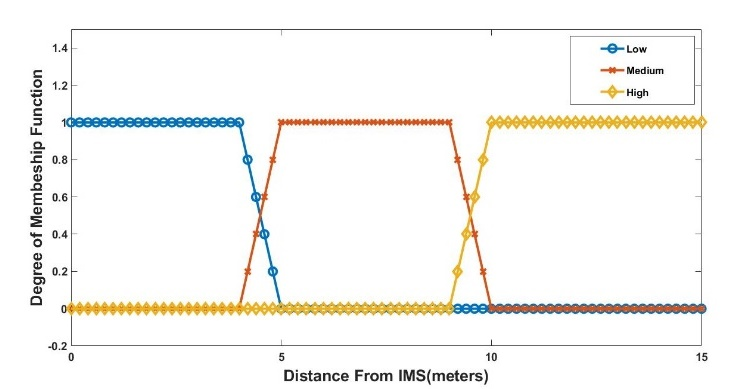In Eq. (7), $\mu _{vd,~ low}\left(vd\right)$ represents the low distance from the IMS.

##### (7)
$\begin{equation} \mu _{vd,low}\left(vd\right)=\left\{\max \left(\min \left(1,\frac{4-vd}{1}\right),0\right)\right\} \end{equation}$

In Eq. (8) $\mu _{vd,medium}\left(vd\right)$ represents the medium-distance membership function from the IMS.

##### (8)
$\begin{equation} \mu _{vd,medium}\left(vd\right)=\left\{\max \left(\min \left(\frac{vd-5}{1},1,\frac{10-vd}{1}\right),0\right)\right\} \end{equation}$

In Eq. (9), $\mu _{vd,high}\left(vd\right)$ represents the high distance from the IMS membership function.

##### (9)
$\begin{equation} \mu _{vd,high}\left(vd\right)=\left\{\max \left(\min \left(\frac{vd-10}{1},1\right),0\right)\right\} \end{equation}$

Fig. 9 shows the graph of the estimated time for a vehicle to reach the IMS, which is divided into three categories. The units used in this membership function are seconds. The line with the "o" marker shows a low-time membership function and ranges from 0-10 seconds. The line with the "x" marker shows the medium time required to reach the IMS, ranging from 8-20 seconds. The line with the "${\lozenge}$" marker shows the high time needed to go to the IMS, ranging from 18-30 seconds. The mathematical form of the membership function is given below.

##### Fig. 9. Estimate time to reach IMS.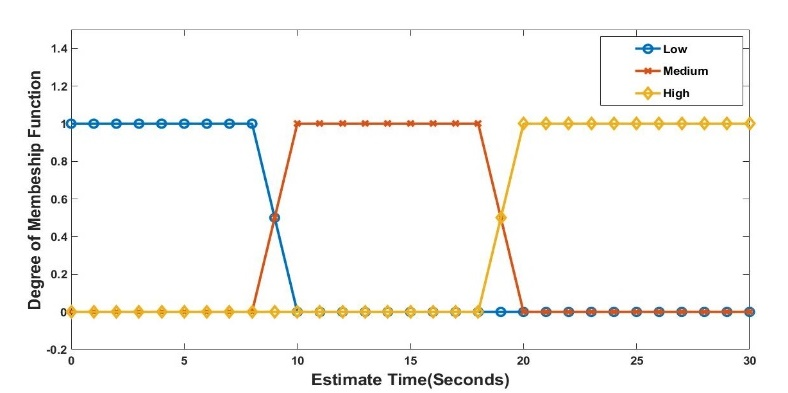In Eq. (10), $\mu _{est,low}\left(et\right)$ shows the low-estimate-time membership function.

##### (10)
$\begin{equation} \mu _{est,low}\left(et\right)=\left\{\max \left(\min \left(1,\frac{8-et}{2}\right),0\right)\right\} \end{equation}$

In Eq. (11), $\mu _{est,medium}\left(est\right)~$represents the membership function for the medium estimate time from the IMS.

##### (11)
$\begin{equation} \mu _{est,medium}\left(et\right)=\left\{\max \left(\min \left(\frac{et-10}{2},1,\frac{20-et}{2}\right),0\right)\right\} \end{equation}$

In Eq. (12), $\mu _{est,high}\left(est\right)$ represents the membership function for the high estimate time to reach the IMS.

##### (12)
$\begin{equation} \mu _{est,high}\left(et\right)=\left\{\max \left(\min \left(\frac{et-20}{2},1\right),0\right)\right\} \end{equation}$

Fig. 10 represents the IMS's output variable at the first layer. The output variable consists of three membership functions. The line with the "o" marker represents the leave membership function. The line with marker "x" represents the standby action by the IMS for the vehicle. The line with the "${\lozenge}$" marker represents the stop membership function.

The mathematical form of the IMS action membership function is given below. In Eq. (13), $\mu _{IMS-action,leave}\left(lve\right)$ represents the leave membership function of the IMS action variable.

##### (13)
$\begin{equation} \mu _{IMS-action,leave}\left(lv\right)~ =\left\{\max \left(\min \left(1,\frac{1-lve}{1}\right),0\right)\right\} \end{equation}$

In Eq. (14), $\mu _{IMS-action}\left(sb\right)$ represents the standby membership function of the IMS action variable.

##### (14)
$\begin{equation} \mu _{IMS-action}\left(sb\right)=\left\{\max \left(\min \left(\frac{sb-2}{1},1,\frac{3-sb}{1}\right),0\right)\right\} \end{equation}$

In Eq. (15), $\mu _{IMS-action}\left(st\right)$ represents the stop membership function of the IMS action variable.

##### (15)
$\begin{equation} \mu _{IMS-action}\left(st\right)=\left\{\max \left(\min \left(\frac{st-4}{1},1\right),0\right)\right\} \end{equation}$

#### 4.1.2 Second Layer

All the IMS output (i.e., IMS1, IMS2, IMS3, and IMS4 output) is fed to the CIMS as input. A graph of individual IMSs is shown in Fig. 10. The mathematical form of all membership functions of the IMSs is given in Eqs. (13) and (14). The CIMS action variable contains four membership functions: Leave-IMS1, Leave-IMS2, Leave-IMS3, and Leave-IMS4. The CIMS finally decides which vehicle should leave the intersection. Fig. 11 shows the graph of output from the CIMS.

##### Fig. 10. IMS output at the first layer.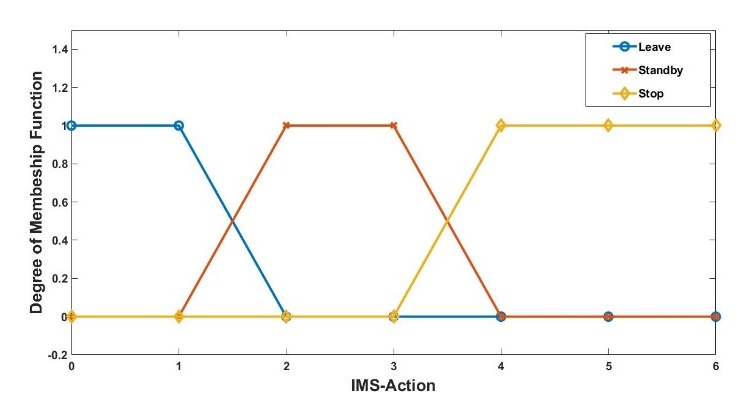These membership functions are expressed mathematically in Eq. (16).

##### (16)
$\begin{equation} \mu _{CIMS,leave-IMS1}\left(lm\right)=\left\{\max \left(\min \left(1,\frac{1-lm}{1}\right),0\right)\right\} \end{equation}$

$\mu _{CIMS,leave-IMS1}\left(lm\right)$ represents the leave-IMS1 membership function.

##### (17)
$\begin{equation*} \mu _{CIMS,leave-IMS2}\left(lm\right)=\left\{\max \left(\min \left(\frac{lm-2}{1},1,\frac{3-lm}{1}\right),0\right)\right\} \end{equation*}$

$\mu _{CIMS,leave-IMS2}\left(lm\right)$ represents the leave-IMS2 membership function.

##### (18)
$\begin{equation*} \mu _{CIMS,leave-IMS3}\left(lm\right)=\left\{\max \left(\min \left(\frac{lm-4}{3},1,\frac{5-lm}{1}\right),0\right)\right\} \end{equation*}$

$\mu _{CIMS,leave-IMS3}\left(lm\right)$ represents the leave-IMS3 membership function.

##### (19)
$\begin{equation} \mu _{CIMS,leave-IMS4}\left(lm\right)=\left\{\max \left(\min \left(\frac{lm-6}{1},1\right),0\right)\right\} \end{equation}$

$\mu _{CIMS,leave-IMS4}\left(lm\right)$ represents the leave-IMS4 membership function.

### 4.2 Fuzzy Propositions

The fuzzy method is constructed using a fuzzy atomic method. The following fuzzy preposition 1 depicts the relation of vehicle lane (vl) and vehicle type (vt) through and connectivity.

FP1=(vl is "Right" and vt is "emergency")

The t-norm function can be defined for the first layer as follows . Eq. (20) transforms the fuzzy membership function of all fuzzy set to the degree of membership to the output of the intersection management system.

##### (20)
$\begin{equation} t_{1}\colon \left[0,1\right]\times \left[0,1\right]\times \left[0,1\right]\times \left[0,1\right]\rightarrow \left[0,1\right] \end{equation}$

For the first layer, this can be expressed as follows. Eq. (21) can be expressed in terms of the t-norm.

##### (21)
 \begin{aligned} &t_{1}\left[\mu_{v l, R}(v l), \mu_{v t}(\text { emergency }), \mu_{v d}(\text { low }), \mu_{e t}(\text { high })\right] \\ &=\min \left[t _ { 1 } \left[\mu_{v l, R}(v l), \mu_{v t, e m e r g e n c y}(v t)\right.\right. \\ &\left., \mu_{v d}(l o w), \mu_{e t}(\text { high })\right] \end{aligned} 
##### (22)
$\begin{equation} \mu _{vl\cap vt\cap vd\cap et}\left(vl,vt,vd,et\right) \\ =t_{1}\left[\mu _{vl}\left(vl\right),\mu _{vt}\left(vt\right),\mu _{vd}\left(vd\right),\mu _{et}\left(et\right)\right] \end{equation}$

By combining Eq. (21) and Eq. (22), Eq. (23) can be written.

##### (23)
\begin{align} \mu _{vl\cap vt\cap vd\cap et}\left(vl,vt,vd,et\right)=\min [t_{1}[\mu _{vl,R}\left(vl\right), \\ ~ \mu _{vt,emergency}\left(vt\right),\mu _{vd}\left(low\right),\mu _{et}\left(high\right)] \end{align}

Similarly, for layer 2, the t-norm function can be defined as follows. Eq. (24) maps the input of the second layer to the fuzzy membership function to the corresponding output membership function. The t-norm function for the second layer can also be defined as :

##### (24)
$\begin{equation} t_{2}\colon \left[0,1\right]\times \left[0,1\right]\times \left[0,1\right]\times \left[0,1\right]\rightarrow \left[0,1\right] \end{equation}$

In Eq. (25), le represents the leave action by IMS1, st represents the stop action by IMS2, sb shows the standby action by IMS3 and IMS4.

##### (25)
\begin{aligned} &t_{2}\left[\mu_{I M S 1}(l e), \mu_{I M S 2}(s t), \mu_{I M S 3}(s b), \mu_{I M S}(s b)\right]= \\ &\min \left[t_{2}\left[\mu_{I M S 1}(l e), \mu_{I M S 2}(s t), \mu_{I M S 3}(s b), \mu_{I M S 4}(s b)\right]\right] \end{aligned}

Eq. (25) can also be defined as:

##### (26)
\begin{aligned} &\mu_{I M S 1} \cap^{I M S 2} \cap{ }^{I M S 3} \cap \cap^{I M S 3}(l e, s t, s b, s b) \\ &=t_{2}\left[\mu_{I M S 1}(l e), \mu_{I M S 2}(s t), \mu_{I M S 3}(s b), \mu_{I M S 4}(s b)\right] \end{aligned}

By combining Eq. (25) and Eq. (26), Eq. (27) can be written .

##### (27)
\begin{aligned} &\mu_{I M S 1} \cap^{I M S 2} \cap \cap^{I M S 3} \cap{ }^{I M S 3}(l e, s t, s b, s b)=\min\\ &\left[t_{2}\left[\mu_{I M S 1}(l e), \mu_{I M S 2}(s t), \mu_{I M S 3}(s b), \mu_{I M S 4}(s b)\right]\right] \end{aligned}

### 4.3 Rules

The rule base is an integral part of the fuzzy system. The rule base provides a realistic scenario of a real-world system. Fuzzy IF-THEN rules are interpreted as fuzzy relation Qlayer1 with a membership function written as :

\begin{equation}
##### (28)
\begin{aligned} &\mu_{Q_{\text {leee } 1}}(v l, v t, v d, e t) \\ &=\min \left[\mu_{F P 1}(v l), \mu_{F P 2}(v t), \mu_{F P 3}(v d), \mu_{F P 4}(e t)\right] \end{aligned}

Similarly, for the second layer, the fuzzy rules can be defined as:

##### (29)
\begin{aligned} &\mu_{Q_{\text {leer } 2}}\left(I M S_{1}, I M S_{2}, I M S_{3}, I M S_{4}\right)= \\ &\min \left[\mu_{F P 1}\left(I M S_{1}\right), \mu_{F P 2}\left(I M S_{2}\right)\right. \\ &\left.\mu_{F P 3}\left(I M S_{3}\right), \mu_{F P 4}\left(I M S_{4}\right)\right] \end{aligned}

A few rules of the first layer are given below.

#### 4.3.1 Rules of First Layer

1. IF (VehicleLane is R) and (VehicleType is EmergencyTrans) and (DistanceFromIMS is Low) and (EstimateTime is Low) THEN (IMSi-action is leave)

2. IF (VehicleLane is R) and (VehicleType is PublicTrans) and (DistanceFromIMS is medium) and (EstimateTime is high) THEN (IMSi-action is standby)

3. IF (VehicleLane is R) and (VehicleType is Pri.Trans) and (DistanceFromIMS is high) and (EstimateTime is high) THEN (IMSi-action is stop)

The total number of rules for the first layer is given below. Rules are denoted by r$^{\mathrm{k}}$.

$Q_{layer1}=\overset{81}{\underset{k=1}\cup }r^{k}$

#### 4.3.2 Rules for Second Layer

1. IF (VehicleLane is R) and (VehicleType is Pri.Trans) and (DistanceFromIMS is high) and (EstimateTime is high) THEN (PrioritytoLeave-IMSi is stop)

2. IF (PrioritytoLeave-IMS1 is standby) and (PrioritytoLeave-IMS2 is leave) and (PrioritytoLeave-IMS3 is standby) and (PrioritytoLeave-IMS4 is standby) THEN (action is leave-ims2)

3. IF (PrioritytoLeave-IMS1 is standby) and (PrioritytoLeave-IMS2 is standby) and (PrioritytoLeave-IMS3 is leave) and (PrioritytoLeave-IMS4 is standby) THEN (action is leave-ims3)

4. IF (PrioritytoLeave-IMS1 is standby) and (PrioritytoLeave-IMS2 is standby) and (PrioritytoLeave-IMS3 is standby) and (PrioritytoLeave-IMS4 is leave) THEN (action is leave-ims4)

The total number of rules for the second layer is given below. Rules are denoted by r$^{\mathrm{m}}$.

$Q_{layer2}=\overset{81}{\underset{m=1}\cup }r^{m}$

### 4.4 Output

The output can be obtained as follows for the first layer .

##### (30)
$\begin{equation} \mu _{vl\cap vt\cap vd\cap et~ }\left(IMS-action\right)=\sup _{I\in \left(vl,vt,vd,et\right)}\\ t\left[\mu _{I}\left(vl,vt,vd,rt\right),\mu _{{Q_{layer1}}}\left(vl,vt,vd,et\right)\right] \end{equation}$
##### (31)
where $Y_{1}=\mu _{out}\left(vl,vt,vd,et\right)$

Mamdani composition-based inference was used. We obtain the product inference engine as :

##### (32)
$\begin{equation} \mu _{out~ }\left(IMS-Action\right)=~ \max _{1\leq I\leq 81}\\ \left[\sup _{I\in \left(vl,vt,vd,et\right)}\left(\prod _{k=1}^{81}\left(\mu _{v{l_{k}},v{t_{k}},v{d_{k}},e{t_{k}}}\left(vl,vt,vd,et\right)\right)\right)\right] \end{equation}$

Similarly, for layer 2, the output can be obtained as follows.

##### (33)
\begin{aligned} &\mu_{I M S_{1} \cap \cap^{I M S_{2}} \cap \cap^{I M S_{3}} \cap{ }^{I M S_{4}}}(C I M S-\text { action })\\ &=\sup _{I \in\left(I M S_{1}, I M S_{2}, I M S_{3}, I M S_{4}\right)} \text { ? }\\ &t\left[\begin{array}{c} \mu_{I}\left(I M S_{1}, I M S_{2}, I M S_{3}, I M S_{4}\right), \\ \mu_{Q_{\text {leer } 2}}\left(\begin{array}{c} I M S_{1} \\ , I M S_{2}, I M S_{3}, I M S_{4} \end{array}\right) \end{array}\right] \end{aligned}
##### (34)
$Y_{2}=\mu _{out}\left(IMS_{1},IMS_{2},IMS_{3},IMS_{4}\right) \\$
##### (35)
\begin{aligned} &\mu_{\text {out }}(C I M S-\text { Action })=\max _{1 \leq I \leq 81} \\ &{\left[\sup _{I \in\left(I M S_{1}, I M S_{2}, I M S_{3}, I M S_{4}\right.}\right.} \\ &\left.\left(\prod_{k=1}^{81}\left(\mu_{I M S 1_{k}, I M S 2_{k}, I M S 3_{k}, I M S} 4_{k}\right)\right)\right] \end{aligned}

### 4.5 Defuzzification

The center of gravity defuzzifier specifies o* as the center of the area covered by a membership function.

##### (36)
$\begin{equation} o^{*}=~ \frac{\int out\mu _{out~ }\left(out\right)dout}{\int \mu _{out~ }\left(out\right)dout~ } \end{equation}$

### 4.6 Functional Comparison with Related Work

Table 5 shows how our proposed work is different from other work that has been done already. In our proposed methodology, the types of vehicles (private, public, and emergency vehicles), the vehicle's speed, and the priority of vehicles (emergency vehicles are always given priority over the other vehicles) are considered. In our proposed system, the conflicts of lanes are also considered. Moreover, our proposed scheme is multi-layer-based, making it a robust prediction system.

##### Table 5. Functional comparative analysis with related work.
 Reference Type of architecture Type of vehicle Speed of vehicle Priority on types of vehicle Focused feature Simulation tool/algorithm  Single, Conflict Matrix No Considered No Reduce average delay time at the intersection SUMO  Multi-level heuristic approach No Considered No Minimum delay SUMO  Mixed-integer programming used for collision avoidance No No No Traffic throughput SUMO  Single-layer No Only flow considered No Traffic control Fuzzy logic + SYNCHRO  Single-layer No No No Traffic Signal Control Fuzzy Logic  Single-layer No No No Traffic congestion Fuzzy + PI controller  Single-layer No No No Traffic Jam Fuzzy logic Proposed Methodology Multi-layered Two-layered Yes Yes Yes Automate intersection Fuzzy logic

## 5. Simulation Results and Discussion

MATLAB 21 was used for simulations. A two-stage-based decision system was used to predict the action taken by the vehicle. This section explains the rule view and surface graph of the proposed systems. Both layers of simulations are discussed in this section.

### 5.1 Rule View

The rule view of the fuzzy logic designer provides the predicted output in the numerical range of the given input in numerical form. The rows shown in Fig. 10 to Fig. 16 are the rules of the proposed model with their inputs and predicted output. A slider line is shown in the figures, which can vary the input value and output changes accordingly.

##### Fig. 12. Fuzzy prediction of IMS action for Leave output.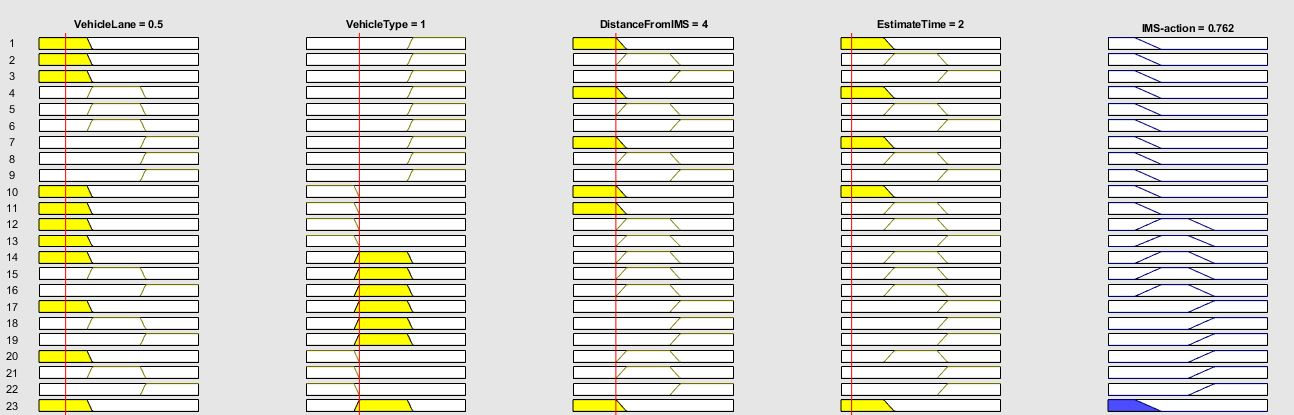##### Fig. 13. Fuzzy prediction of the IMS action for Standby output.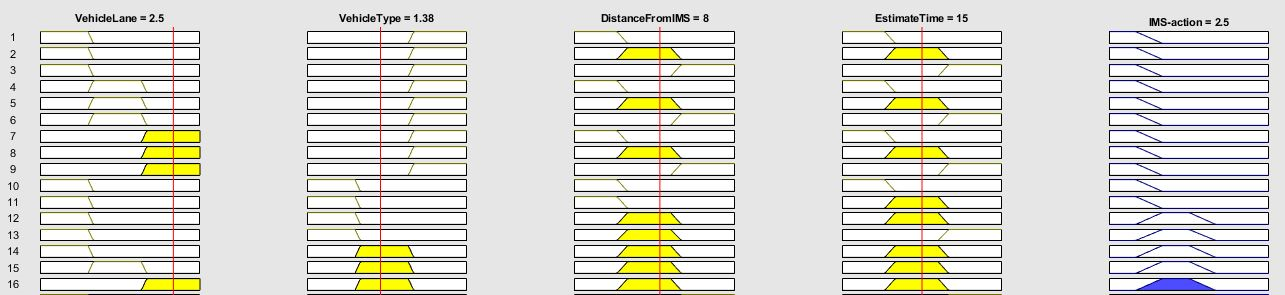##### Fig. 14. Fuzzy prediction of IMS for Stop output.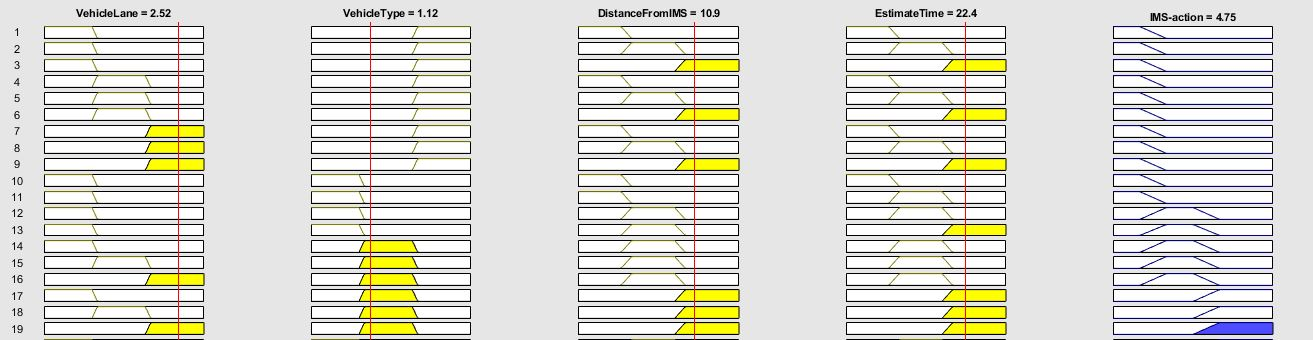##### Fig. 15. Fuzzy prediction of CIMS for Leave-IMS1 output.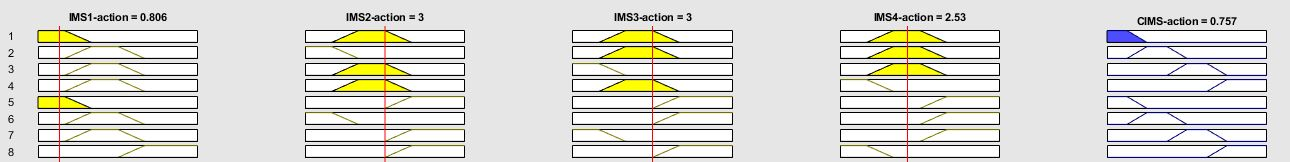##### Fig. 16. Fuzzy prediction of CIMS for Leave-IMS2 output.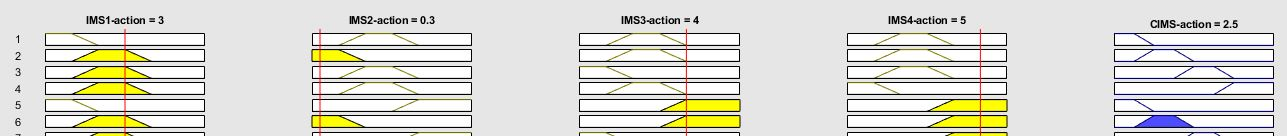#### 5.1.1 Rule View for the First Layer

It is shown in Fig. 12 that if VehicleLane is Right (0.5), VehileType is Private Transport (1), DistanceFromIMS is Low (4), and EstimateTime is Low (2), then the IMS action is Leave (0.762).

In Fig. 13, it is shown that if VehicleLane is Left (2.5), VehileType is Private Transport (1.38), DistanceFromIMS is Medium (8), and EstimateTime is Medium (15), then the IMS action is Standby (2.5).

It is shown in Fig. 14 that if VehicleLane is Left (2.52), VehileType is Private Transport (1.12), DistanceFromIMS is High (10.9), and EstimateTime is High (22.4), then the IMS action is Stop (4.75).

#### 5.1.2 Rule View for the Second Layer

Similarly, the rule view for the second layer also predicts the output based on inputs given to the system. The output of the first layer is fed as inputs of the second layer. In Fig. 15, it is shown that if IMS1 action is Leave (0.806), IMS2 action is Standby (3), IMS3 action is Standby (3), and IMS4 action is Standby (2.53), then the CIMS output is leave-IMS1 (0.757).

In Fig. 16, it is depicted that if IMS1 action is Standby (3), IMS2 action is Leave (0.3), IMS3 action is Stop (4), and IMS4 action is Stop (5), then the CIMS output is leave-IMS2 (2.5).

In Fig. 17, it is shown that if IMS1 action is Standby (60%), the stop (40%) value is 3.39, IMS2 action is Stop (5.34), IMS3 action is Leave (0.913), and IMS4 action is Stop (5.01), then the CIMS output is leave-IMS3 (4.5).

In Fig. 18, it is shown that if IMS1 action is Standby (3), IMS2 action is Stop (5),, IMS3 action is Standby (3), and IMS4 action is Leave (0.2), then the CIMS output is leave-IMS4 (6.75).

##### Fig. 17. Fuzzy prediction of CIMS for Leave-IMS3 output.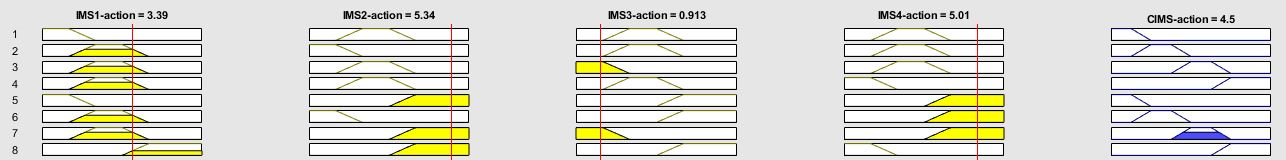##### Fig. 18. Fuzzy prediction of CIMS for Leave IMS4 output.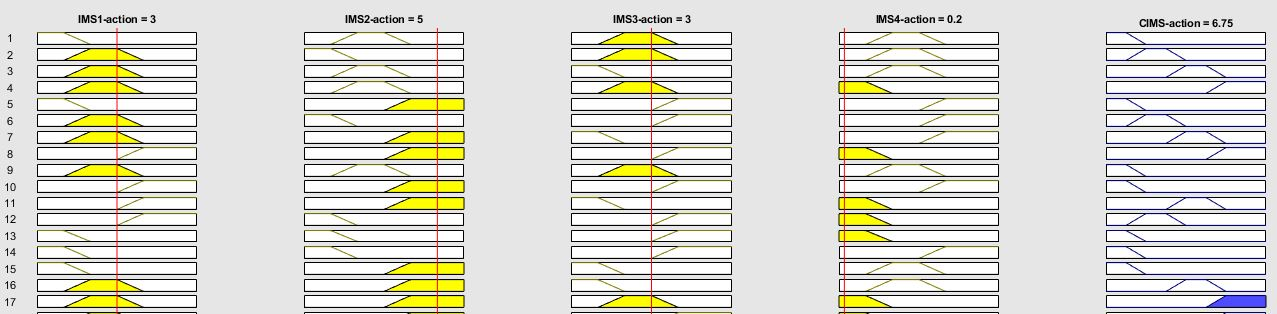### 5.2 Surface Graphs

In a fuzzy inference system, the surface graph shows the total area covered by all inputs and their resulting output. The surface graph shows all the variations of the system.

#### 5.2.1 Selected Surface Graph of the First Layer

The surface graph of the IMS action concerning VehicleLane and VehicleType is shown in Fig. 19. In Fig. 19, the x-axis is the VehicleLane ranging from 0 to 3. On the y-axis, the VehicleType variable is shown and ranges from 0 to 3. On the z-axis, the output is shown in terms of the IMS action. In Fig. 19, the blue region represents the leave action by the IMS. The blue region lies in all vehicle lanes, but VehicleType ranges from 2-3. The yellow region is 0-1 or 1-2 for VehicleLane and VehicleType. The yellow region represents the chances of stop (70%) and standby (30%)actions. According to Fig. 19, if VehicleLane is Right and VehicleType is public transport, then the resulting region is blue, which allows the vehicle to leave. But when VehicleLane is the center lane and VehicleType is private transport, then the resulting region is yellow. In the yellow region, the vehicle does a standby action. The rest of the area of the surface is green, which shows standby action.

Fig. 20 shows the surface graph of the IMS action on the z-axis concerning the variable DistanceFromIMS on the x-axis and the variable EstimateTime on the y-axis. In Fig. 20, the blue region lies in the DistanceFromIMS range of 0-5 (low distance), EstimateTime is 0-5, and we use the low-estimate-time membership function. For the blue region, the vehicle receives an action to leave the IMS.

##### Fig. 19. Surface of the first layer between the vehicle type and vehicle lane variable.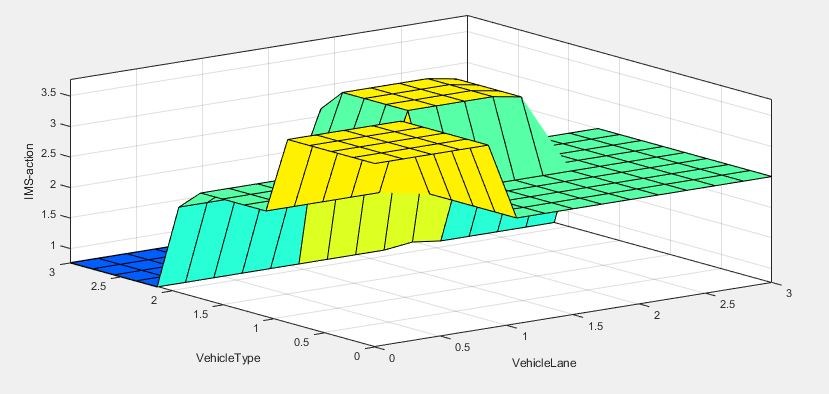##### Fig. 20. Surface of the first layer between the DistanceFromIMS and EstimateTime variables.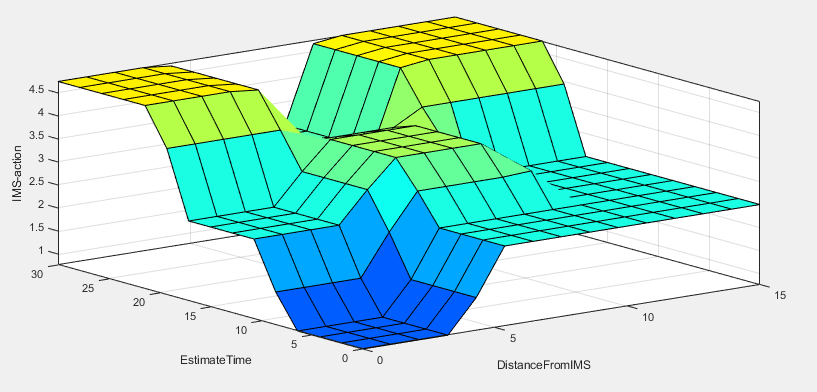##### Fig. 21. Surface graph between the IMS1 action and IMS2 action.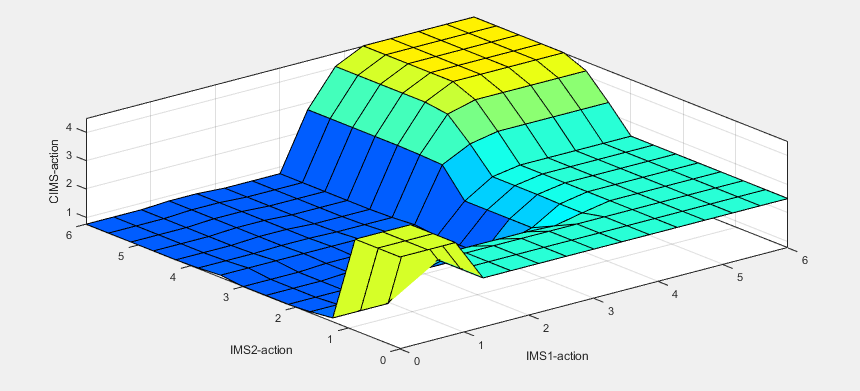The DistanceFromIMS is 0-5 (low distance) and 10-15 (medium distance) for the yellow region. The EstimateTime (20-30) is a high time required to reach the IMS. In the yellow area, the vehicle receives a stop action. Similarly, for the range of medium EstimateTime, the vehicle receives a stop action. The remaining surface lies in the sea-green region related to the standby action. The light green region lies in the medium ranges of DistanceFromIMS and EstimateTime. The variation from standby to stop is observed in the light green region.

#### 5.2.2 Selected Surface Graph for the Second Layer

Fig. 21 shows the surface variation of the CIMS action for IMS1 action and IMS2 action. On the x-axis, the variable IMS1 action is shown, and on the y-axis, the IMS2 action variable is shown. The z-axis contains the output of the CIMS action variable. In the surface graph, the yellow region lies in the range of 0-1 for IMS1, 0-1 for IMS2, and 4-6 for both IMS1 action and IMS2 action. In the yellow region, the vehicle receives an action that says IMS1's vehicle can leave. It is important to note that the surface observed here is made by fixing IMS3 and IMS4 to a value of 5.

The yellow region can be explained based on the rules of a fuzzy inference system. If IMS1 action is leave, IMS2 action is leave or standby, IMS3 action is stop, and IMS4 action is stop, the fuzzy inference system prioritizes IMS1's vehicle to leave. The range of 4-6 for IMS1 and IMS2 shows a conflict case. In case of conflict, the fuzzy logic controller prioritizes IMS1. This is due to all vehicles being on standby or stopping. Then, the CIMS will allow vehicles in round fashion starting from IMS1 to IMS4. The blue region lies in the IMS1 ranges of 1.5 to 3.5 and 2-6. The CIMS makes IMS1 and IMS2 vehicles stop in this region and lets the IMS3 vehicle move. The rest of the sea-green region also makes IMS1 and IMS2 vehicles stop.

Fig. 22 shows the surface graph of the CIMS action, IMS3 action, and IMS4 action. On the x-axis is the IMS3 action, and on the y-axis is the IMS4 action. On the z-axis, the CIMS action is shown, which is the system's output. For the surface, IMS1 and IMS2 are given stop action by fixing the value to 5. This surface graph also contains yellow, blue, green, and sea-green regions. The yellow region lies in the range of 2-6 for the IMS3 action and 0-1.5 for the IMS4 action. The yellow region shows that if the IMS3 action is stop and the IMS4 action is leave, the CIMS predict the leave action for IMS4's vehicle.

The blue region lies in the range of 2-4 for the IMS4 action and 2-6 for the IMS3 action. In the blue region, if the IMS3 action's vehicle is standby or stop and the IMS4 action's vehicle has the same status, then the fuzzy inference system prioritizes the IMS3 action's vehicle. The green region lies in the range of 0-1 for IMS3 and 0-3 for IMS4. Another region that is also green lies in the range of 2-6 for IMS3 action and 4-6 for IMS4 action. For the first green region, the CIMS allows IMS3's vehicle to leave. For the second green region, the CIMS prioritizes IMS1 as all the IMS's vehicles are stopped. The sea-green region lies in the range of 0-1.5 for IMS3 action and 4-6 for IMS4 action. The CIMS allows IMS3's vehicle to leave in the sea-green region, as IMS4's vehicle is on standby or stopped.

##### Fig. 22. Surface graph between the IMS3 action and IMS4 action.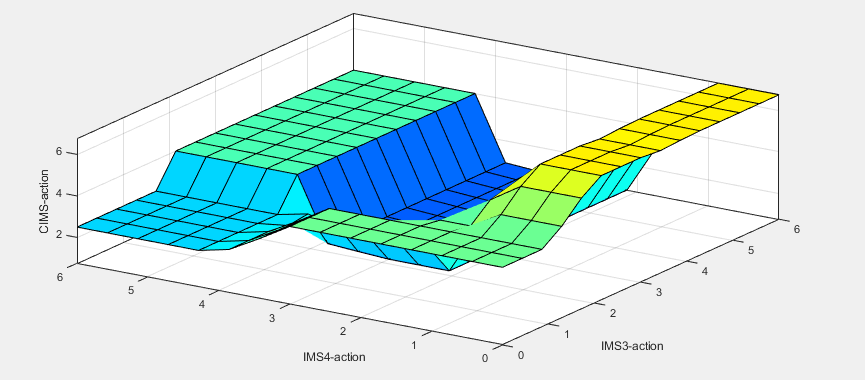## 4. Conclusion

An autonomous decision system is a key element for implementing future applications. The proposed model provides a solution for an IMS based on vehicle-to-infrastructure communication. Accident avoidance is the key motivation for the IMS. Our proposed strategy is different from other work due to consideration of types of vehicles and the proposed fuzzy-based controller. The proposed approach is also better in terms of the central controlling body. The vehicle has to share only a few parameters. Fuzzy logic is also a better candidate in terms of computational complexity.

While most of the previous research in the literature is static, the method proposed in this paper is dynamic with the change of the traffic environment. As the vehicle leaves the safety zone, its connection with the IMS and the CIMS is lost. This feature also does not put too much burden on the controlling unit. The controlling mechanism can be shifted to a distributed system for future work, in which vehicles can share their public information of routing with other vehicles. A smaller decision body can be implemented in vehicles, making the proposed system more robust and autonomous. In the future, it is also recommended that all intersection controlling bodies be able to communicate with each other to establish a complete network of a vehicle to infrastructure.

### ACKNOWLEDGMENTS

This work was supported by the National Research Foundation of Korea (NRF) grant, which is funded by the Korean government (MSIT) (No. 2021R1F1A105089311).

### REFERENCES

1
Chen Feng, Song Mingtao, Ma Xiaoxiang, 2019, Investigation on the Injury Severity of Drivers in Rear-End Collisions Between Cars Using a Random Parameters Bivariate Ordered Probit Model, International Journal of Environmental Research and Public Health, Vol. 16, No. 26322
Kawser Mohammad, Sajjad Syed, Fahad Saymon, Ahmed Sakib, Rafi Hasib, 2019, The Perspective of Vehicle-to-Everything (V2X) Communication towards 5G, Vol. 19, pp. 146-155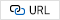3
Haider Malik Amir, Hwang Seung-Hoon, 2019, Adaptive Transmit Power Control Algorithm for Sensing-Based Semi-Persistent Scheduling in C-V2X Mode 4 Communication, Electronics, Vol. 8, No. 8464
Li Yuying, Liu Qipeng, 2020, Intersection management for autonomous vehicles with vehicle-to-infrastructure communication, PLOS ONE, Vol. 15, No. e02356445
Parasumanna Gokulan Balaji, Srinivasan D, 2010, Multi-Agent System in Urban Traffic Signal Control, Computational Intelligence Magazine, IEEE, Vol. 5, pp. 43-516
Hamidi H, Kamankesh A, 2018, An Approach to Intelligent Traffic Management System Using a Multi-agent System, Int. J. ITS Res., Vol. 16, pp. 112-1247
Chouhan. A. P, Banda G, 2018, Autonomous Intersection Management: A Heuristic Approach, in IEEE Access, Vol. 6, pp. 53287-532958
Lu Qiang, Kim Kyoung-Dae, 2018, A Mixed Integer Programming Approach for Autonomous and Connected Intersection Crossing Traffic Control9
AlNaser Nada B, Hawas Yaser E, 2019, A Traffic Signal Controller for an Isolated Intersection using Fuzzy Logic Model10
Chen. L, Englund C, Feb. 2016, Cooperative Intersection Management: A Survey, in IEEE Transactions on Intelligent Transportation Systems, Vol. 17, No. 2, pp. 570-58611
Mohanaselvi. S, Shanpriya B, 2019, Application of fuzzy logic to control traffic signals, AIP Conference Proceedings 2112, Vol. 02004512
Milan Koukol , Lenka Zajíčková , Lukáš Marek , Pavel Tuček , 2015, Fuzzy Logic in Traffic Engineering: A Review on Signal Control, Mathematical Problems in Engineering, Vol. 2015, No. article id 979160, pp. 1413
Tunc Ilhan, Söylemez Mehmet, April, 27-30 2020, Intelligent Intersection Management using Fuzzy Logic Control and PI control, Proceedings of 8th Transport Research Arena TRA 2020, Helsinki, Finland14
Babangida Zachariah , Peter Ayuba. , Luhutyit Peter Damuut , 2017, OPTIMIZATION OF TRAFFIC LIGHT CONTROL SYSTEM OF AN INTERSECTION USING FUZZY INFERENCE SYSTEM, Science World Journal, Vol. 12, No. 4, pp. 27-3315
Namazi E, Li. J, Lu C, 2019, Intelligent Intersection Management Systems Considering Autonomous Vehicles: A Systematic Literature Review, in IEEE Access, Vol. 7, pp. 91946-9196516
Perera L. P, Carvalho. J. P, Guedes Soares C, May 2014, Solutions to the Failures and Limitations of Mamdani Fuzzy Inference in Ship Navigation, in IEEE Transactions on Vehicular Technology, Vol. 63, No. 4, pp. 1539-155417
Sang-Woong Lee , Sadaf Hussain , Ghassan F. Issa , Sagheer Abbas , Taher M. Ghazal , Tanweer Sohail , Munir Ahmad , 2021, Multi-dimensional Trust Quantification by Artificial Agents through Evidential Fuzzy Multi-Criteria Decision Making., IEEE Access, Vol. 9, pp. 159399-15941218
Sadaf Hussain , Rizwan Ali Naqvi , Sagheer Abbas , Muhammad Adnan Khan , Tanweer Sohail. , 2021, Trait Based Trustworthiness Assessment in Human-Agent Collaboration Using Multi-Layer Fuzzy Inference Approach., IEEE Access 9, pp. 73561-7357419
Kashif Iqbal , Muhammad Adnan , Sagheer Abbas , Zahid Hasan , 2018, Intelligent Transportation System (ITS) for Smart-Cities using Mamdani Fuzzy Inference System., International Journal of Advanced Computer Science and Applications, Vol. 9, No. 220
Gulzar Ahmad , Muhammad Adnan Khan , Sagheer Abbas , Atifa Athar , Bilal Shoaib Khan , 2019, Automated diagnosis of hepatitis b using multilayer mamdani fuzzy inference system., Journal of healthcare engineering21
Areej Fatima , Muhammad Adnan Khan , Sagheer Abbas , Muhammad Waqas , Leena Anum , 2019, Evaluation of planet factors of smart city through multi-layer fuzzy logic (MFL)., The ISC International Journal of Information Security, Vol. 11, No. 3, pp. 51-5822
Sadaf Hussain , Sagheer Abbas , Tanweer Sohail , M. Adnan Khan , 2019, Estimating virtual trust of cognitive agents using multi layered socio-fuzzy inference system., Journal of Intelligent., Fuzzy Systems, Vol. 37, No. 2, pp. 2769-2784## Author

Engr. Muhammad Nadeem Ali is currently pursuing a Ph.D. at Hongik University, South Korea. He completed his BS and MS in Electronics Engineering from International Islamic University Islamabad in 2012 and 2016 respectively. His BS in Electronics Engineering was fully funded by Ministry of Information and Technology, Government of Pakistan for four year. The scholarship name was ICT & RD Fund. Currently, he is member of Broadband Convergence Network Laboratory in Hongik University. His major interests are 5G wireless communication system, intelligent transport system and its application, Latency issues in networks, edge computing, and name data network. From 2015 to 2019, he was working as Senior Lecture at Department of Computer Science, Lahore Garrison University. Where he supervised many final year projects. He was also Assistant Editor of Lahore Garrison University Journal of Computer Science and Information Technology during 2017 to 2019. He also member of review panel at 3rd International Conference on Innovative Computing (ICIC) 2021 organized by University Management and Technology.

Byung-Seo Kin received his B.S degree in electrical engineering from in-Ha University, In-Chon, Korea, in 1998 and his M.S and PhD degrees in electrical and computer engineering from the University of Florida in 2001 and 2004, respectively. His PhD study was supervised by Dr Yuguang Fang between 1997 and 1999, he worked for Motorola Korea Ltd., PAJU Korea, as a computer integrated manufacturing (CIM) engineer in advanced technology research and development (ATR&D) from January 2005 to August 2007, and he worked for Motorola Inc., Schaumburg Illinois, as a senior software engineer in network and enterprise. His research in Motorola Inc. was designing a protocol and network architecture for wireless broadband mission-critical communication. From 2012 to 2014, he was the chairman of the software and communication engineering department at Hongik University, Korea, where he is currently a professor. He is a senior IEEE member and serves as an associate editor of IEEE Access, Telecommunication system, and the journal of the Institute of electrics and information engineers. He also served as guest editor of the special issue of IEEE internet of things, IEEE Access, the international journal of the distributed sensor network (SAGE), sensors, and applied science. His work has appeared in around 240 publications and 32 patents. His research interests include designing and developing efficient wireless/wired networks, including link-adaptable/cross-layer-based protocols, multi-protocol structure, wireless CCNs/NDNs, mobile edge computing, and physical layer design for broadband PLC resource allocation algorithms for wireless networks.